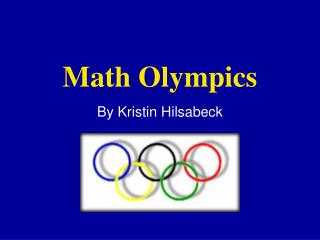DownloadDownload PresentationMath Olympics

# Math Olympics

Download Presentation## Math Olympics

- - - - - - - - - - - - - - - - - - - - - - - - - - - E N D - - - - - - - - - - - - - - - - - - - - - - - - - - -
##### Presentation Transcript

1. Math Olympics By Kristin Hilsabeck

2. Math Olympics Have students create a math game that reviews past or chapter material. In a list of requirements, include specific topics and areas you want the students to review.

3. Math Scavenger Hunt Students must solve the problem or equation at the bottom of the paper. Once solved, the students find the answer on the top of a different sheet of paper then solve the question at the bottom. Students must solve all problems until they return to the equation were they started.

4. Rules For Math Scavenger Hunt • Equations are placed around the classroom • Students must show all work in order to receive full credit • Students can work in pairs • Students must go in order of the equations

5. Topics That Must Be Included In This Project • Parametric Equations • Trigonometric Equations • Matrices • Natural Logs

6. Example M,n,mn,mn 1 kjlkjljlk 5.4931 mnm Write the parametric equation for the line 9x + y = -1 The determinant of -1 4 is 2 -3 5 x = t. y = -9t - 1 Evaluate the expression ln 243 Simplify the equation cos x csc x tan x

7. Variations of Math Scavenger Hunt • Combine math and physical education and have the answer include some type of activity in the elementary level. • For example: Q: 4 x 2 = A: 8 jumping jacks

8. Grading Students can be graded on the following in Math Olympics: • Creativity • Degree of Difficulty • Participation • Following Directions • Presentation

9. Extra Grades You can use the activities the students present in your lesson plans through out the year and count them as extra homework or quiz grades

10. TEKS for Math Olympics Basically any of the TEKS can be covered in this project depending on your specific requirements for the topic areas.